Reach Us+44-1522-440391
A class of unitary irreducible representations of the Lie superalgebra osp(1|2n) 1 | OMICS International
Journal of Generalized Lie Theory and Applications
All submissions of the EM system will be redirected to Online Manuscript Submission System. Authors are requested to submit articles directly to Online Manuscript Submission System of respective journal.

# A class of unitary irreducible representations of the Lie superalgebra osp(1|2n) 1

Stijn LIEVENS*, Nedialka I. STOILOVA and Joris Van der JEUGT

Department of Applied Mathematics and Computer Science, Ghent University, Krijgslaan 281-S9, B-9000 Gent, Belgium

*Corresponding Author:
Stijn LIEVENS
E-mail:
[email protected], [email protected], [email protected]

Received date: December 14, 2007; Revised date: March 08, 2008

Visit for more related articles at Journal of Generalized Lie Theory and Applications

#### Abstract

Using the equivalence of the defining relations of the orthosymplectic Lie superalgebra osp(1|2n) to the defining triple relations of n pairs of parabose operators b± i we construct a class of unitary irreducible (infinite-dimensional) lowest weight representations V (p) of osp(1|2n). We introduce an orthogonal basis of V (p) in terms of Gelfand-Zetlin patterns, where the subalgebra u(n) of osp(1|2n) plays a crucial role and we present explicit actions of the osp(1|2n) generators.

Following some physical ideas we construct a class of infinite dimensional unitary irreducible representations of the Lie superalgebra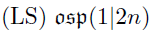in an explicit form. In 1953 Green  introduced the so called parabose operators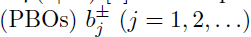satisfying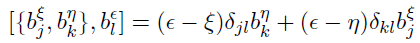(1)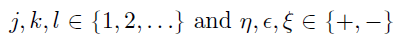(to be interpreted as +1 and −1 in the algebraic expressions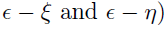as a generalization of the ordinary Bose operators. The Fock space V (p) of n pairs of PBOs is a Hilbert space with a vacuum |0>, defined by means of (j, k = 1, 2, . . . , n)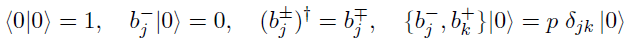(2)

and by irreducibility under the action of the algebra spanned by the elements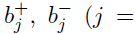1, . . . , n), subject to (1). The parameter p is referred to as the order of the paraboson system. However the structure of the parabose Fock space is not known, also a proper basis has not been introduced. We solve these problems using the relation between n pairs of PBOs and the defining relations of the LS osp(1|2n), discovered by Ganchev and Palev . The orthosymplectic superalgebra osp(1|2n)  consists of matrices of the form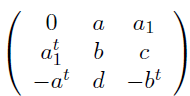(3)

where a and a1 are (1 × n)-matrices, b is any (n × n)-matrix, and c and d are symmetric (n × n)-matrices. The even elements have a = a1 = 0 and the odd elements are those with b = c = d = 0. Denote the row and column indices running from 0 to 2n and by eij the matrix with zeros everywhere except a 1 on position (i, j). Then as a basis in the Cartan subalgebra h of osp(1|2n) consider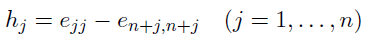(4)

In terms of the dual basis ±j of h*, the root vectors and corresponding roots of osp(1|2n) are given by: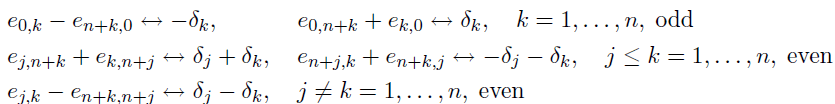Introduce the following multiples of the odd root vectors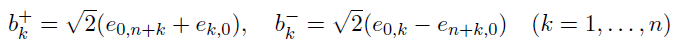(5)

Then the following holds :

Theorem 1 (Ganchev and Palev). As a Lie superalgebra defined by generators and relations, osp(1|2n) is generated by 2n odd elements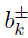subject to the parabose relations (1).

From (4) and (5) it follows that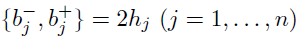and using (2) we have:

Corollary 2. The parabose Fock space V (p) is the unitary irreducible representation of osp(1|2n) with lowest weight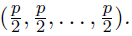We can construct the representation V (p)  using an induced module construction with an appropriate chain of subalgebras.

Proposition 3. A basis for the even subalgebra sp(2n) of osp(1|2n) is given by the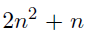elements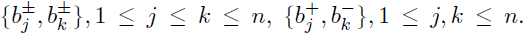The n2 elements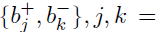1, . . . , n are a basis for the sp(2n) subalgebra u(n).

The subalgebra u(n) can be extended to a parabolic subalgebra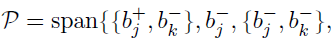j, k = 1, . . . , n}  of osp(1|2n). Recall that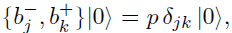with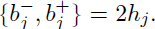Then the space spanned by |0i is a trivial one-dimensional u(n) module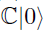of weight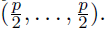Since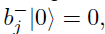the modulecan be extended to a one-dimensional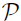module. Now we define the induced osp(1|2n) module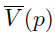with lowest weight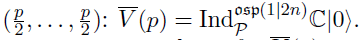By the Poincar´e-Birkhoff-Witt theorem [1,4], it is easy to give a basis for.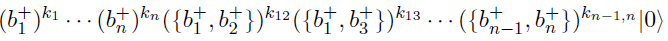where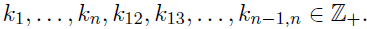However in generalis not a simple module and let M(p) be the maximal nontrivial submodule of. Then the simple module (irreducible module), corresponding to the paraboson Fock space, is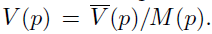The purpose is now to determine the vectors belonging to M(p) and also to find explicit matrix elements of the osp(1|2n) generators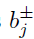in an appropriate basis of V (p).

From the basis init is easy to write down the character of: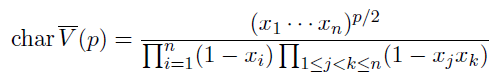(6) .

Such expressions have an interesting expansion in terms of Schur functions.

Proposition 4 (Cauchy, Littlewood). Let x1, . . . , xn be a set of n variables. Then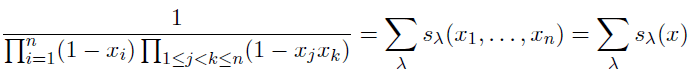(7)

Herein the sum is over all partitions ¸ and sλ(x) is the Schur symmetric function .

The characters of finite dimensional u(n) representations are given by such Schur functions s¸(x). For such finite dimensional u(n) representations labelled by a partition ¸, there is a known basis: the Gelfand-Zetlin basis (GZ) . We shall use the u(n) GZ basis vectors as our new basis for. Thus the new basis ofconsists of vectors of the form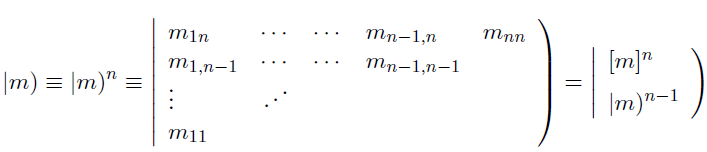(8)

where the top line of the pattern, also denoted by the n-tuple [m]n , is any partition ¸ (consisting of non increasing nonnegative numbers) with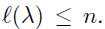The label p itself is dropped in the notation of |m). The remaining n−1 lines of the pattern will sometimes be denoted by |m)n−1. So all mij in the above GZ-pattern are nonnegative integers, satisfying the betweenness conditions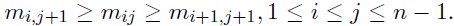Note that, since the weight of |0> is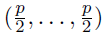, the weight of the above vector is determined by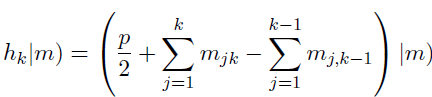(9)

The triple relations (1), imply that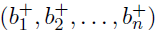is a standard u(n) tensor of rank (1, 0, . . . , 0). Therefore we can attach a unique GZ-pattern with top line 10 · · · 0 to every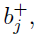corresponding to the weight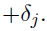. Explicitly: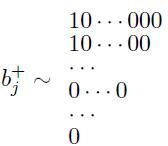(10)

where the pattern consists of j − 1 zero rows at the bottom, and the first n − j + 1 rows are of the form 10 · · · 0. The tensor product rule in u(n) reads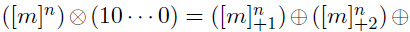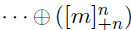where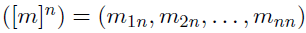and a subscript ±k indicates an increment of the kth label by ±1: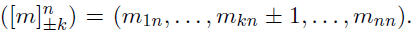A general matrix element ofcan now be written as follows: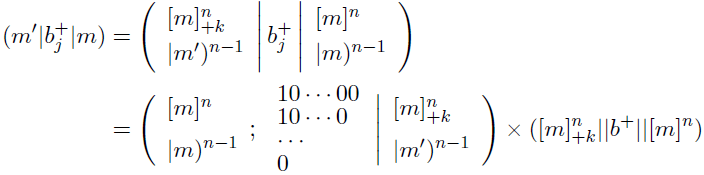The first factor in the right hand side is a u(n) Clebsch-Gordan coefficient , the second factor is a reduced matrix element. By the tensor product rule, the first line of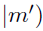has to be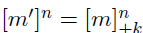for some k-value.

The special u(n) CGCs appearing here are well known, and have fairly simple expressions. They can be found, e.g. in [8,9]. The actual problem is now converted into finding expressions for the reduced matrix elements, i.e. for the functions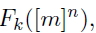for arbitrary n-tuples of non increasing nonnegative integers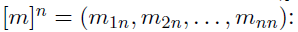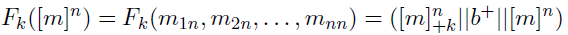(11)

So one can write: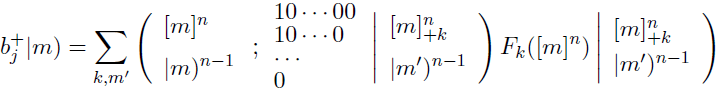(12)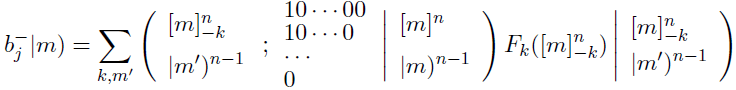(13)

For j = n, the CGCs in (12)-(13) take a simple form , and one has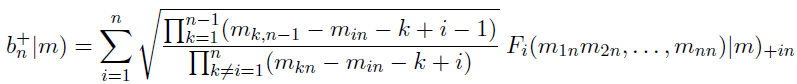(14)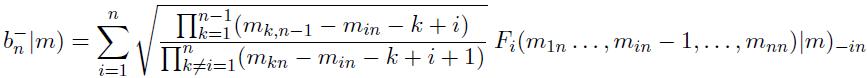(15)

In order to determine the n unknown functions Fk, one can start from the following action: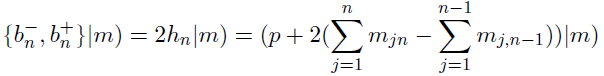(16)

Expressing the left hand side by means of (14)–(15), one finds a system of coupled recurrence relations for the functions Fk. Taking the appropriate boundary conditions into account, we have been able to solve this system of relations .

Proposition 5. The reduced matrix elements Fk appearing in the actions ofon vectors |m) of V (p) are given by: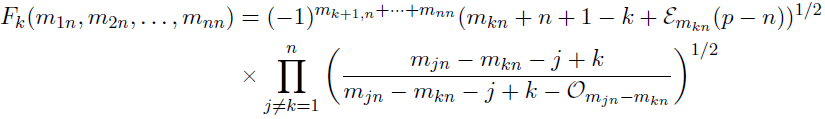(17)

where ε and O are the even and odd functions defined by εj = 1 if j is even and 0 otherwise, Oj = 1 if j is odd and 0 otherwise.

The proof consists of verifying that all triple relations (1) hold when acting on any vector |m). Each such verification leads to an algebraic identity in n variables m1n, . . . ,mnn. In these computations, there are some intermediate verifications: e.g the action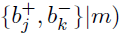should leave the top row of the GZ-pattern |m) invariant (since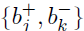belongs to u(n)). Furthermore, it must yield (up to a factor 2) the known action of the standard u(n) matrix elements Ejk in the classical GZ-basis. Consider now the factor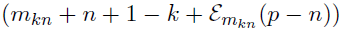in the expression of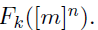This is the only factor in the right hand side of (17) that may become zero. If this factor is zero or negative, the assigned vector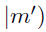belongs to M(p). Recall that the integers mjn satisfy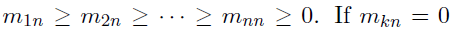(its smallest possible value), then this factor in Fk takes the value (p−k+1). So the p-values 1, 2, . . . , n−1 play a special role leading to the following result :

Theorem 6. The osp(1|2n) representation V (p) with lowest weight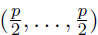is a unirrep if and only if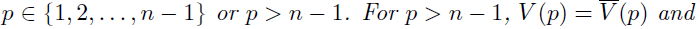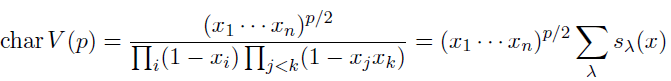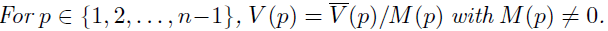The structure of V (p) is determined by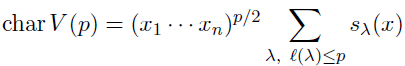where `(¸) is the length of the partition λ.

The explicit action of the osp(1|2n) generators in V (p) is given by (12)-(13), and the basis is orthogonal and normalized. For p 2 {1, 2, . . . , n − 1} this action remains valid, provided one keeps in mind that all vectors with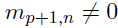must vanish.

Note that the first line of Theorem 6 can also be deduced from [10,11], where all unirreps of osp(1|2n) are classified.

#### Acknowledgements

N. I. Stoilova was supported by a project from the Fund for Scientific Research – Flanders (Belgium) and by project P6/02 of the Interuniversity Attraction Poles Programme (Belgian State – Belgian Science Policy). S. Lievens was also supported by project P6/02.

#### References

Select your language of interest to view the total content in your interested language

### Article Usage

• Total views: 11703
• [From(publication date):
September-2008 - Aug 19, 2019]
• Breakdown by view type
• HTML page views : 7926
• PDF downloads : 3777

## Post your commentCan't read the image? click here to refresh
###### Peer Reviewed Journals

Make the best use of Scientific Research and information from our 700 + peer reviewed, Open Access Journals

International Conferences 2019-20

Meet Inspiring Speakers and Experts at our 3000+ Global Annual Meetings

Top